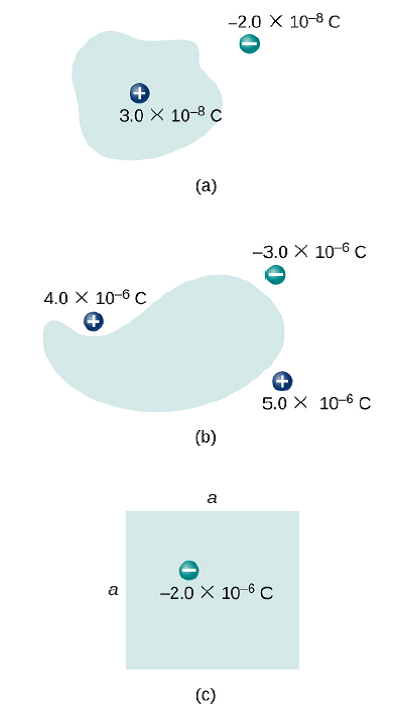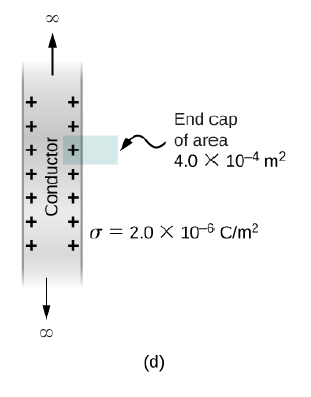# Problem: Find the electric flux through the closed surface whose cross sections are shown below.

###### FREE Expert Solution

Electric flux:

$\overline{){{\mathbf{\varphi }}}_{{\mathbf{E}}}{\mathbf{=}}\frac{{\mathbf{Q}}_{\mathbf{enc}}}{{\mathbf{\epsilon }}_{\mathbf{0}}}}$, where Q is the electric charge enclosed in the given cross-sectional area and ε0 is the permittivity in free space.

(a)

ΦE = (3.0 × 10-8)/(8.85 × 10-12) = 3389.8 Nm2/C

The electric flux through the closed surface whose cross-section is shown in figure (a) is 3389.8 Nm2/C.

81% (450 ratings)###### Problem Details

Find the electric flux through the closed surface whose cross sections are shown below.